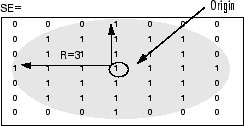# strel

## 说明

`strel` 对象表示一个平面形态学结构元素，该元素是形态学膨胀和腐蚀运算的重要部分。## 创建对象

### 语法

``SE = strel(nhood)``
``SE = strel("diamond",r)``
``SE = strel("disk",r)``
``SE = strel("disk",r,n)``
``SE = strel("octagon",r)``
``SE = strel("line",len,deg)``
``SE = strel("rectangle",[m n])``
``SE = strel("square",w)``
``SE = strel("cube",w)``
``SE = strel("cuboid",[m n p])``
``SE = strel("sphere",r)``

### 描述

#### 任意邻域形状

``SE = strel(nhood)` 创建一个具有指定邻域 `nhood` 的平面结构元素。`

#### 二维几何邻域形状

``SE = strel("diamond",r)` 创建一个菱形结构元素，其中 `r` 指定从结构元素原点到菱形各点的距离。`

``SE = strel("disk",r)` 创建一个盘形结构元素，其中 `r` 指定半径。`
``SE = strel("disk",r,n)` 创建一个盘形结构元素，其中 `r` 指定半径，`n` 指定用于逼近盘形的线条结构元素的数量。当结构元素使用逼近时，使用盘形逼近的形态学运算的运行速度要快得多。`
``SE = strel("octagon",r)` 创建一个八边形结构元素，其中 `r` 指定从结构元素原点到八边形边的距离，沿水平和垂直轴测量。`r` 必须为 3 的非负倍数。`

``SE = strel("line",len,deg)` 创建一个关于邻域中心对称的线性结构元素，长度约为 `len`，角度约为 `deg`。`
``SE = strel("rectangle",[m n])` 创建一个大小为 `[m n]` 的矩形结构元素。`

``SE = strel("square",w)` 创建一个宽度为 `w` 个像素的正方形结构元素。`

#### 三维几何邻域形状

``SE = strel("cube",w)` 创建一个宽度为 `w` 个像素的三维立方体结构元素。`
``SE = strel("cuboid",[m n p])` 创建一个大小为 m×n×p 像素的三维立方体结构元素。 `

``SE = strel("sphere",r)` 创建一个半径为 `r` 个像素的三维球面结构元素。`

• `SE = strel("arbitrary",nhood,h)`，其中 `h` 是与 `nhood` 大小相同的矩阵，包含着与 `nhood` 的每个非零元素相关联的高度值。

• `SE = strel("ball",r,h,n)`

### 输入参数

• 对于盘形，`r` 是从原点到盘边的距离。

• 对于菱形，`r` 是从结构元素原点到菱形各点的距离。

• 对于八边形，`r` 是从结构元素原点到八边形边的距离，沿水平和垂直轴测量。`r` 必须为 3 的倍数。

• 对于球面形状，`r` 是从原点到球面的距离。

n 的值行为
`n` > 0`strel` 使用包含 `n` 个循环线条形结构元素的序列来逼近形状。有时 `strel` 必须在逼近中使用两个额外的线条结构元素，在这种情况下，分解结构元素的实际数量是 `n+2`
`n` = 0`strel` 不使用任何逼近。结构元素成员包括中心距原点不大于 `r` 的所有像素。

## 对象函数

 `imdilate` 膨胀图像 `imerode` 腐蚀图像 `imclose` 对图像执行形态学闭运算 `imopen` 对图像执行形态学开运算 `imbothat` Bottom-hat filtering `imtophat` Top-hat filtering `bwhitmiss` Binary hit-miss operation
 `decompose` Return sequence of decomposed structuring elements `reflect` Reflect structuring element `translate` Translate structuring element

## 示例

`SE = strel('square', 11)`
```SE = strel is a square shaped structuring element with properties: Neighborhood: [11x11 logical] Dimensionality: 2 ```

`SE = strel('line', 10, 45)`
```SE = strel is a line shaped structuring element with properties: Neighborhood: [7x7 logical] Dimensionality: 2 ```

`SE.Neighborhood`
```ans = 7x7 logical array 0 0 0 0 0 0 1 0 0 0 0 0 1 0 0 0 0 0 1 0 0 0 0 0 1 0 0 0 0 0 1 0 0 0 0 0 1 0 0 0 0 0 1 0 0 0 0 0 0 ```

`SE3 = strel('disk', 15)`
```SE3 = strel is a disk shaped structuring element with properties: Neighborhood: [29x29 logical] Dimensionality: 2 ```

```figure imshow(SE3.Neighborhood)````SE = strel('sphere', 15)`
```SE = strel is a sphere shaped structuring element with properties: Neighborhood: [31x31x31 logical] Dimensionality: 3 ```

```figure isosurface(SE.Neighborhood)```## 提示

• 不使用逼近 (`n` = 0) 的结构元素不适合计算粒度。

## 算法

 van den Boomgard, R, and R. van Balen, "Methods for Fast Morphological Image Transforms Using Bitmapped Images," Computer Vision, Graphics, and Image Processing: Graphical Models and Image Processing, Vol. 54, Number 3, pp. 252–254, May 1992.

 Adams, R., "Radial Decomposition of Discs and Spheres," Computer Vision, Graphics, and Image Processing: Graphical Models and Image Processing, Vol. 55, Number 5, pp. 325–332, September 1993.

 Jones, R., and P. Soille, "Periodic lines: Definition, cascades, and application to granulometrie," Pattern Recognition Letters, Vol. 17, pp. 1057–1063, 1996.